# Methods and formulas for the model summary in Fit Model and Discover Key Predictors with TreeNet® Classification

###### Note

Choose the method or formula of your choice.

## Important predictors

The number of predictors with positive relative importance.
A TreeNet® Classification model comes from a sequence of small regression trees that use generalized residuals as the response variable. The calculation of the model improvement score for a predictor from a single tree has two steps:
1. Find the reduction in mean squared errors when the predictor splits a node.
2. Add all the reductions from all the nodes where the predictor is the node splitter.

Then, the importance score for the predictor equals the sum of the model improvement scores across all the trees.

## Average –loglikelihood for a binary response

The calculations depend on the validation method.

where

and

### Notation for training data or no validation

TermDescription
Nsample size of the full or training data set
wiweight for the ith observation in the full or training data set
yiith response value that is 1 for the event and 0 otherwise for the full or training data set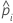predicted probability of the event for the ith row in the full or training data set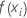fitted value from the model

where

and

### Notation for k-fold cross-validation

TermDescription
Nsample size of the full or training data
nksample size of fold k
wi, kweight for the ith observation in fold k
yi, kbinary response value of case i in fold k. yi, k = 1 for event class, and 0 otherwise.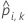predicted probability for case i in fold k. The predicted probability is from the model that does not use the data in fold k.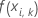fitted value for case i in fold k. The fitted value is from the model that does not use the data in fold k.

where

and

### Notation for test data set

TermDescription
nTestsample size of the test data set
wi, Testweight for the ith observation in the test data set
yi, Testbinary response value of case i in fold k in the test data set. yi, k = 1 for event class, and 0 otherwise.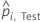predicted probability for case i in the test data set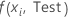fitted value for case i in the test data set

## Average –loglikelihood for a multinomial response

The calculations depend on the validation method. In the following sections,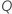is the number of levels in the response variable.

where

### Notation for training data or no validation

TermDescription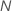sample size of the full or training data set
wiweight for the ith observation in the full or training data set
yi, qith response value that is 1 when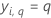and 0 otherwise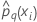predicted probability of the qth level of the response for the ith row in the full or training data set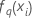fitted value from the qth sequence of trees for the ith row, which is used to calculate the predicted probability of the qth level of the response

where

### Notation for k-fold cross-validation

TermDescription
Nsample size of the training data
nksample size of fold k
wi, kweight for the ith observation in fold k
yi, k, qith response value of case i in fold k that is 1 when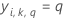and 0 otherwise.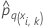The predicted probability of the qth level of the response for the ith row in fold k. The predicted probability is from the model that does not use the data in fold k.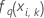The fitted value from the qth sequence of trees for the ith row in fold k, which is used to calculate the predicted probability of the qth level of the response. The fitted value is from the model that does not use the data in fold k.

where

### Notation for test data set

TermDescription
nTestsample size of the test data
wi, Testweight for the ith observation in the test data
yi, Test, qith response value of case i in the test data set that is 1 when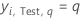and 0 otherwise.The predicted probability of the qth level of the response for the ith row in the test data. The predicted probability is from the model that does not use the test data.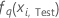The fitted value for the qth sequence of trees for the ith row in the test data, which is used to calculate the predicted probability of the qth level of the response. The predicted probability is from the model that does not use the test data.

## Area under ROC curve

The Model Summary table includes the area under the ROC curve when the response is binary. The ROC curve plots the true positive rate (TPR), also known as power, on the y-axis, and the false positive rate (FPR), also known as type 1 error, on the x-axis. The area under the ROC curve values typically range from 0.5 to 1.

### Formula

The area under the curve is a summation of areas of trapezoids:

where k is the number of distinct event probabilities and (x0, y0) is the point (0, 0).

To compute the area for a curve from a test data set or from cross-validated data, use the points from the corresponding curve.

### Notation

TermDescription
TPR true positive rate
FPR false positive rate
TPtrue positive, events that were correctly assessed
FNfalse negative, events that were incorrectly assessed
P number of actual positive events
FPfalse positive, nonevents that were incorrectly assessed
N number of actual negative events
FNRfalse negative rate
TNRtrue negative rate

### Example

For example, suppose your results have 4 distinct fitted values with the following coordinates on the ROC curve:
x (false positive rate) y (true positive rate)
0.0923 0.3051
0.4154 0.7288
0.7538 0.9322
1 1
Then the area under the ROC curve is given by the following calculation: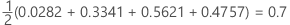## 95% CI for the area under the ROC curve

Minitab calculates a confidence interval for the area under the Receiver Operating Characteristic curve when the response is binary.

The following interval gives the upper and lower bounds for the confidence interval:

The computation of the standard error of the area under the ROC curve (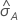) comes from Salford Predictive Modeler®. For general information about estimation of the variance of the area under the ROC curve, see the following references:

Engelmann, B. (2011). Measures of a ratings discriminative power: Applications and limitations. In B. Engelmann & R. Rauhmeier (Eds.), The Basel II Risk Parameters: Estimation, Validation, Stress Testing - With Applications to Loan Risk Management (2nd ed.) Heidelberg; New York: Springer. doi:10.1007/978-3-642-16114-8

Cortes, C. and Mohri, M. (2005). Confidence intervals for the area under the ROC curve. Advances in neural information processing systems, 305-312.

Feng, D., Cortese, G., & Baumgartner, R. (2017). A comparison of confidence/credible interval methods for the area under the ROC curve for continuous diagnostic tests with small sample size. Statistical Methods in Medical Research, 26(6), 2603-2621. doi:10.1177/0962280215602040

### Notation

TermDescription
Aarea under the ROC curve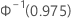0.975 percentile of the standard normal distribution

## Lift

Minitab displays lift in the model summary table when the response is binary. The lift in the model summary table is the cumulative lift for 10% of the data.

## Misclassification rate

In the weighted case, use weighted counts in place of counts.

For k-fold cross-validation, the misclassed count is the sum of the misclassifications from when each fold is the test data set.

For validation with a test data set, the misclassed count is the sum of misclassifications in the test data set and the total count is for the test data set.

By using this site you agree to the use of cookies for analytics and personalized content.  Read our policy